# NCERT Solutions For Class 6 Maths Decimals Exercise 8.6

ncert textbook

## NCERT Solutions For Class 6 Maths Decimals Exercise 8.6

NCERT Solutions for Class 6 Maths Chapter 8 Decimals Ex 8.6

Exercise 8.6

Question 1.
Subtract:
(a) ₹18.25 from ₹20.75
(b) 202.54 m from 250 m
(c) ₹5.36 from ₹8.40
(d) 2.051 km from 5.206 km
(e) 0.314 kg from 2.107 kg
Solution:
(a) ₹18.25 from 20.75
= 20.75 – 18.25
= 2.50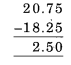(b) 202.54 m from 250 m
= 250 m – 202.54 m
= 250.00 m – 202.54 m
= 47.46 m(c) ₹5.36 from ₹8.40
= ₹8.40 – ₹5.36
= ₹3.04(d) 2.051 km from 5.206 km
= 5.206 km – 2.051 km
= 3.155 km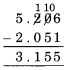(e) 0.314 kg from 2.107 kg
= 2.107 kg – 0.314 kg
= 1.793 kgQuestion 2.
Find the value of:
(a) 9.756 – 6.28
(b) 21.05 – 15.27
(c) 18.5 – 6.79
(d) 11.6 – 9.847
Solution:
(a) We have 9.756 – 6.25
= 9.756 – 6.250
= 3.506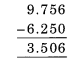(b) We have 21.05 – 15.27
= 5.78(c) We have 18.5 – 6.79
= 18.50 – 6.79
= 11.71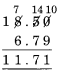(d) 11.6 – 9.847
= 11.600 – 9.847
= 1.753Question 3.
Raju bought a book for ₹35.65. He gave ₹50 to the shopkeeper. How much money did he get back from the shopkeeper?
Solution:
Cost of book = ₹35.65
Money paid by him to the shopkeeper = ₹50
∴ Money got back by him
= ₹50 – ₹35.65
= ₹50.00 – ₹35.65
= ₹14.35Question 4.
Rani had ₹18.50. She bought one ice-cream for ₹11.75. How much money does she have now?
Solution:
She bought ice-cream for ₹11.75
∴ Money left with Rani = ₹ 18.50 – ₹11.75 = ₹6.75Question 5.
Tina had 20 m 5 cm long cloth. She cuts 4 m 50 cm length of cloth from this for making a curtain. How much cloth is left with her?
Solution:
Length of cloth had by Tina = 20 m 5 cm = 20.05 m
Length of cloth cut by her = 4 m 50 cm
= 4.50 m
∴ Length of cloth left with her = 20.05 m -4.50 m
= 15.55 m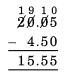Question 6.
Namita travels 20 km 50 m every day. Out of this she travels 10 km 200 m by bus and the rest by auto. How much distance does she travel by auto?
Solution:
Distance travelled by Namita daily = 20 km 50 m or 20.050 km
Distance travelled by her by bus = 10 km 200 m or 10.200 km
∴ Distance travelled by her by auto = (20.050 – 10.200) km = 9.850 kmQuestion 7.
Aakash bought vegetables weighing 10 kg. Out of this, 3 kg 500 g is onions, 2 kg 75 g is tomatoes and the rest is potatoes. What is the weight of the potatoes?
Solution:
Weight of vegetables bought by Aakash = 10 kg
Weight of onions bought by him = 3 kg 500 g
= 3.500 kg
and weight of tomatoes bought by him = 2 kg 75 g = 2.075 kg
∴ Weight of potatoes = Weight of vegetable – (weight of onions + weight of tomatoes)
= 10.000 – (3.500 + 2.075)
= 10.000 – 5.575 = 4.425 kg